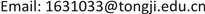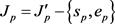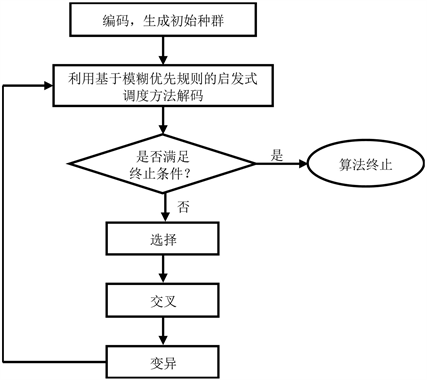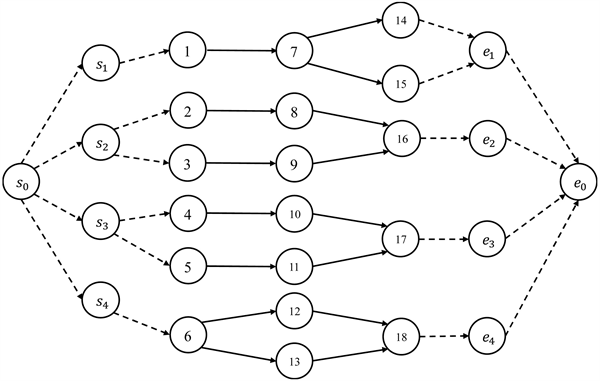﻿ 考虑资源转移成本的模糊资源受限多项目调度研究 Fuzzy Resource-Constrained Multi-Project Scheduling Problem with Resource Transfer Cost

Operations Research and Fuzziology
Vol. 09  No. 01 ( 2019 ), Article ID: 28631 , 9 pages
10.12677/ORF.2019.91005

Fuzzy Resource-Constrained Multi-Project Scheduling Problem with Resource Transfer Cost

Mingxiao Zhang

School of Economics and Management, Tongji University, ShanghaiReceived: Jan. 4th, 2019; accepted: Jan. 18th, 2019; published: Jan. 25th, 2019ABSTRACT

Based on the traditional resource-constrained multi-project scheduling model, this paper considered resource transfer time and cost, and used triangular fuzzy numbers to express activity duration and resource transfer time to describe the uncertainties in the actual scheduling process. While making up for the shortcomings of previous studies, it is closer to the reality and has certain guiding significance for multi-project management. In the solution algorithm, the common genetic algorithm is adopted, and the corresponding fuzzy priority rules are given for the multi-project scheduling model with limited resources considering the cost of resource transfer. After data experiments, the genetic algorithm based on the fuzzy priority rules can solve the proposed model well.

Keywords:Project Scheduling, Triangular Fuzzy Number, Resource Transfer, Genetic Algorithm1. 引言

2. 考虑资源转移成本的模糊资源受限多项目调度问题

2.1. 问题描述

1) 工序一旦开始不得被打断；

2) 所讨论的资源均为可更新资源，比如施工机械等，且工序所需资源必须从其他工序处转移，资源转移所需的时间 $\stackrel{˜}{{\Delta }_{ijr}}$ 仅由工序i、工序j和资源r的类型决定，与资源转移数量无关。

3) 资源在同一项目内部转移不需要耗费时间和成本，仅在不同项目间转移需要耗费时间和成本，且转移时间 $\stackrel{˜}{{\Delta }_{ijr}}$ 和转移成本 $\stackrel{˜}{{C}_{ijr}}$ 均满足三角不等式，即 $\stackrel{˜}{{\Delta }_{ijr}}+\stackrel{˜}{{\Delta }_{hjr}}>\stackrel{˜}{{\Delta }_{ijr}}$$\stackrel{˜}{{C}_{ijr}}+\stackrel{˜}{{C}_{hjr}}>\stackrel{˜}{{C}_{ijr}}$

2.2. 三角模糊数

$\stackrel{˜}{{d}_{i}}+\stackrel{˜}{{d}_{j}}=\left({d}_{i}^{L}+{d}_{j}^{L},{d}_{i}^{M}+{d}_{j}^{M},{d}_{i}^{U}+{d}_{j}^{U}\right)$ (2-1)

$\stackrel{˜}{{d}_{i}}-\stackrel{˜}{{d}_{j}}=\left({d}_{i}^{L}-{d}_{j}^{U},{d}_{i}^{M}-{d}_{j}^{M},{d}_{i}^{U}-{d}_{j}^{L}\right)$ (2-2)

$\stackrel{˜}{{d}_{i}}×\stackrel{˜}{{d}_{j}}=\left({d}_{i}^{L}×{d}_{j}^{L},{d}_{i}^{M}×{d}_{j}^{M},{d}_{i}^{U}×{d}_{j}^{U}\right)$ (2-3)

$\stackrel{˜}{{d}_{i}}/\stackrel{˜}{{d}_{j}}=\left({d}_{i}^{L}/{d}_{j}^{U},{d}_{i}^{M}/{d}_{j}^{M},{d}_{i}^{U}/{d}_{j}^{L}\right)$ (2-4)

$\stackrel{˜}{{d}_{i}^{-1}}=\left(1/{d}_{i}^{U},1/{d}_{i}^{M},1/{d}_{i}^{L}\right)$ (2-5)

${\mathrm{log}}_{n}\left(\stackrel{˜}{{d}_{i}}\right)=\left({\mathrm{log}}_{n}\left({d}_{i}^{L}\right),{\mathrm{log}}_{n}\left({d}_{i}^{M}\right),{\mathrm{log}}_{n}\left({d}_{i}^{U}\right)\right)$ (2-6)

$\stackrel{˜}{{d}_{i}}+a=\left({d}_{i}^{L}+a,{d}_{i}^{M}+a,{d}_{i}^{U}+a\right)$ (2-7)

$\stackrel{˜}{{d}_{i}}-a=\left({d}_{i}^{L}-a,{d}_{i}^{M}-a,{d}_{i}^{U}-a\right)$ (2-8)

$\stackrel{˜}{{d}_{i}}×a=\left({d}_{i}^{L}×a,{d}_{i}^{M}×a,{d}_{i}^{U}×a\right)$ (2-9)

$\stackrel{˜}{{d}_{i}}/a=\left({d}_{i}^{L}/a,{d}_{i}^{M}/a,{d}_{i}^{U}/a\right)$ (2-10)

$a/\stackrel{˜}{{d}_{j}}=\left(a/{d}_{j}^{U},a/{d}_{j}^{M},a/{d}_{j}^{L}\right)$ (2-11)

$I\left(\stackrel{˜}{{d}_{i}}\right)=\frac{{d}_{i}^{L}+2{d}_{i}^{M}+{d}_{i}^{U}}{4}$ (2-12)

1) 若 $I\left(\stackrel{˜}{{d}_{i}}\right) ，则称 $\stackrel{˜}{{d}_{i}}<\stackrel{˜}{{d}_{j}}$

2) 若 $I\left(\stackrel{˜}{{d}_{i}}\right)=I\left(\stackrel{˜}{{d}_{j}}\right)$ ，则称 $\stackrel{˜}{{d}_{i}}=\stackrel{˜}{{d}_{j}}$

3) 若 $I\left(\stackrel{˜}{{d}_{i}}\right)>I\left(\stackrel{˜}{{d}_{j}}\right)$ ，则称 $\stackrel{˜}{{d}_{i}}>\stackrel{˜}{{d}_{j}}$

2.3. 数学模型

1) 模型相关符号及变量说明：

$P$ 多项目调度问题的所有项目集合， $P=\left\{{p}_{1},{p}_{2},\cdots ,{p}_{m}\right\}$

${s}_{0},{e}_{0}$ 多项目调度问题整体的虚拟开始工序和虚拟结束工序；

${s}_{p},{e}_{p}$ 项目p的虚拟开始工序和虚拟结束工序；

${{J}^{\prime }}_{p}$ 项目p的所有工序集合；

${J}_{p}$ 项目p的所有实工序集合，${J}^{\prime }$ 多项目调度问题所有项目的工序集合， ${J}^{\prime }=\left\{1,2,\cdots ,n\right\}$

$J$ 多项目调度问题所有项目的实工序集合， $J={J}^{\prime }-\left\{{s}_{0},{e}_{0}\right\}-{\cup }_{p\in P}\left\{{s}_{p},{e}_{p}\right\}$

$\stackrel{˜}{{d}_{j}}$ 工序j的持续时间， $\stackrel{˜}{{d}_{j}}=\left({d}_{j}^{L},{d}_{j}^{M},{d}_{j}^{U}\right)$

$\stackrel{˜}{{\Delta }_{ijr}}$ 资源r从工序i转移到工序j所需的时间， $\stackrel{˜}{{\Delta }_{ijr}}=\left({\Delta }_{ijr}^{L},{\Delta }_{ijr}^{M},{\Delta }_{ijr}^{U}\right)$

t， $T$ 时间索引，多项目调度问题工期的上界；

$\stackrel{˜}{{F}_{i}}$ 工序j的完工时间；

${A}_{j}$ 工序j的直接紧前工序集合， ${A}_{{s}_{0}}=\varnothing$${A}_{{e}_{0}}={\cap }_{p\in P}\left\{{e}_{p}\right\}$${A}_{{s}_{p}}=\left\{{s}_{0}\right\}$

${A}_{j}^{\text{*}}$ 工序j的直接和间接紧前工序集合；

${S}_{j}$ 工序j的直接紧后工序集合， ${S}_{{e}_{0}}=\varnothing$${S}_{{s}_{0}}={\cap }_{p\in P}\left\{{s}_{p}\right\}$${S}_{{e}_{p}}=\left\{{e}_{0}\right\}$

${S}_{j}^{\text{*}}$ 工序j的直接和间接紧后工序集合；

$r$$R$ 资源索引，多项目调度问题的所有资源集合；

${a}_{r}$ 资源r的数量；

${u}_{jr}$ 工序j所需资源r的数量， ${u}_{{s}_{0}r}={u}_{{e}_{0}r}={a}_{r},{u}_{{s}_{p}r}={u}_{{e}_{p}r}=0,\forall r\in R$

${I}_{{r}_{j}}$ 可以为任务j转移资源r的工序集合 ${I}_{{r}_{j}}=JU\left\{{s}_{0}\right\}-\left\{j\right\}-{S}_{j}^{*}$

${O}_{{r}_{j}}$ 工序j可以传递资源r的工序集合 ${O}_{{r}_{j}}=JU\left\{{e}_{0}\right\}-\left\{j\right\}-{A}_{j}^{*}$

$C{P}_{p}$ 项目p的合同期限；

${T}_{p}$ 项目p的单位拖期成本(或奖励)；

${C}_{ijr}$ 资源r从工序i转移到工序j的单位成本；

$M$ 无穷大的参数；

${f}_{jt}$ 如果任务j在t时刻开始则为1，否则为0；

${N}_{ijr}$ 资源r从工序i转移到工序j的数量；

${x}_{ijr}$ 如果资源r从工序i转移到工序j则为1，否则为0；

2) 数学模型：

$\mathrm{min}\stackrel{˜}{MC}={\sum }_{p\in P}\left(\stackrel{˜}{{F}_{{e}_{p}}}-C{P}_{p}\right)\cdot {T}_{p}+{\sum }^{\text{​}}{N}_{ijr}\cdot {C}_{ijr}\cdot {x}_{ijr}$ (2-13)

 (2-14)

$\stackrel{˜}{{F}_{j}}={\sum }_{t=0}^{T-\stackrel{˜}{{d}_{j}}}\left(t+\stackrel{˜}{{d}_{j}}\right)\cdot {f}_{jt},\forall j\in J$ (2-15)

$\stackrel{˜}{{F}_{j}}-\stackrel{˜}{{F}_{i}}\ge \stackrel{˜}{{d}_{j}}\forall j\in J,\forall i\in {I}_{{r}_{j}}$ (2-16)(2-17)

${N}_{ijr}\le {x}_{ijr}\cdot \mathrm{min}\left\{{u}_{ir},{u}_{jr}\right\}\forall j\in J,\forall i\in {I}_{{r}_{j}}\forall r\in R$ (2-18)

${x}_{ijr}\le {N}_{ijr}\forall j\in J,\forall i\in {I}_{{r}_{j}},\forall r\in R$ (2-19)

${\sum }_{i\in {I}_{{r}_{j}}}{N}_{ijr}={u}_{jr}\forall j\in J,\forall r\in R$ (2-20)

${\sum }_{j\in {O}_{{r}_{i}}}{N}_{ijr}={u}_{ir}\forall i\in J,\forall r\in R$ (2-21)

${\sum }_{j=1}^{n}{\sum }_{\tau =t}^{t+\stackrel{˜}{{d}_{j}}}{u}_{jr}\cdot {f}_{j\tau }\le {a}_{r}\forall t\in T,\forall r\in R$ (2-22)

${f}_{jt}\in \left(0,1\right),{x}_{ijr}\in \left(0,1\right),{N}_{ijr}\ge 0,\forall j\in J,\forall i\in J,\forall r\in R,\forall t\in T$ (2-23)

3. 基于模糊优先规则的遗传算法

3.1. 模糊优先规则

3.2. 遗传算法设计

1) 编码

2) 解码

3) 适应值函数

4) 交叉和变异

4. 数值实验Figure 1. The flow of genetic algorithms based on fuzzy priority rulesFigure 2. The network diagram of multi-project scheduling examples

Continued

Continued

5. 结论与展望

Fuzzy Resource-Constrained Multi-Project Scheduling Problem with Resource Transfer Cost[J]. 运筹与模糊学, 2019, 09(01): 36-44. https://doi.org/10.12677/ORF.2019.91005

1. 1. Yan, Y., Kuphal, T. and Bode, J. (2000) Application of Multi-Agent System in Project Management. International Journal of Production Economics, 68, 185-197. https://doi.org/10.1016/S0925-5273(00)00082-7

2. 2. Kelly, J.E. (1963) The Critical Path Method: Resource Planning and Scheduling. Prentice-Hall, Englewood Cliffs, 347-365.

3. 3. Payne, J.H. (1995) Management of Multiple Simultaneous Projects: A State-of-the-Art Review. Inter-national Journal of Project Management, 13, 163-168. https://doi.org/10.1016/0263-7863(94)00019-9

4. 4. Fendley, L.G. (1968) Towards the Development of a Complete Multi-Project Scheduling System. Journal of Industrial Engineering, 19, 505-515.

5. 5. Kruger, D. and Scholl, A. (2009) A Heuristic Solution Framework for the Resource Constrained (Multi-)Project Scheduling Problem with Se-quence-Dependent Transfer Times. European Journal of Operational Research, 197, 492-508. https://doi.org/10.1016/j.ejor.2008.07.036

6. 6. 宗砚, 刘琼, 张超勇, 朱海平. 考虑资源传递时间的多项目调度问题[J]. 计算机集成制造系统, 2011, 17(9): 1921-1928.

7. 7. 郭云涛, 陈志, 白思俊. 转移资源受限多项目调度的改进量子遗传算法[J]. 工业工程与管理, 2014(3): 33-39.

8. 8. Prade, H. (1979) Using Fuzzy Set Theory in a Scheduling Problem: A Case Study. Fuzzy Sets and Systems, 2, 153-165. https://doi.org/10.1016/0165-0114(79)90022-8

9. 9. 孙若昕. 基于优先规则的模糊资源受限多项目调度研究[D]: [硕士学位论文]. 天津: 天津大学, 2012.